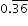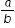# MAFS.8.NS.1.1Archived StandardExport Print
Know that numbers that are not rational are called irrational. Understand informally that every number has a decimal expansion; for rational numbers show that the decimal expansion repeats eventually, and convert a decimal expansion which repeats eventually into a rational number.
General Information
Subject Area: Mathematics
Domain-Subdomain: The Number System
Cluster: Level 1: Recall
Cluster: Know that there are numbers that are not rational, and approximate them by rational numbers. (Supporting Cluster) -

Clusters should not be sorted from Major to Supporting and then taught in that order. To do so would strip the coherence of the mathematical ideas and miss the opportunity to enhance the major work of the grade with the supporting clusters.

Date of Last Rating: 02/14
Status: State Board Approved - Archived
Assessed: Yes
Test Item Specifications

• Assessment Limits :
All irrational numbers may be used, excluding ??. Only rational numbers with repeating decimal expansions up to thousandths may be used.
• Calculator :

No

• Context :

No context

Sample Test Items (4)

• Test Item #: Sample Item 3
• Question:

What iswritten as a fraction?

• Difficulty: N/A
• Type: EE: Equation Editor

## Related Courses

This benchmark is part of these courses.
1205050: M/J Accelerated Mathematics Grade 7 (Specifically in versions: 2014 - 2015, 2015 - 2020, 2020 - 2022, 2022 and beyond (current))
1205070: M/J Grade 8 Pre-Algebra (Specifically in versions: 2014 - 2015, 2015 - 2022, 2022 and beyond (current))
1204000: M/J Foundational Skills in Mathematics 6-8 (Specifically in versions: 2014 - 2015, 2015 - 2022, 2022 and beyond (current))
1200410: Mathematics for College Success (Specifically in versions: 2014 - 2015, 2015 - 2022 (course terminated))
1200700: Mathematics for College Algebra (Specifically in versions: 2014 - 2015, 2015 - 2022 (course terminated))
7812030: Access M/J Grade 8 Pre-Algebra (Specifically in versions: 2014 - 2015, 2015 - 2018, 2018 - 2019, 2019 - 2022, 2022 and beyond (current))
7912115: Fundamental Explorations in Mathematics 2 (Specifically in versions: 2013 - 2015, 2015 - 2017 (course terminated))

## Related Access Points

Alternate version of this benchmark for students with significant cognitive disabilities.

## Related Resources

Vetted resources educators can use to teach the concepts and skills in this benchmark.

## Formative Assessments

Repeating Decimals:

This lesson unit is intended to help you assess how well students are able to:

• Translate between decimal and fraction notation, particularly when the decimals are repeating.
• Create and solve simple linear equations to find the fractional equivalent of a repeating decimal.
• Understand the effect of multiplying a decimal by a power of 10.

Type: Formative Assessment

Rational Numbers:

Students are asked to identify rational numbers from a list of real numbers, explain how to identify rational numbers, and to identify the number system that contains numbers that are not rational.

Type: Formative Assessment

Decimal to Fraction Conversion:

Students are given several terminating and repeating decimals and asked to convert them to fractions.

Type: Formative Assessment

Fraction to Decimal Conversion:

Students are given a fraction to convert to a decimal and are asked to determine if the decimal repeats.

Type: Formative Assessment

## Lesson Plans

Repeating Decimals:

This lesson unit is intended to help you assess how well students are able to translate between decimal and fraction notation, particularly when the decimals are repeating, create and solve simple linear equations to find the fractional equivalent of a repeating decimal, and understand the effect of multiplying a decimal by a power of 10.

Type: Lesson Plan

Who's Being Irrational?!:

In this lesson, students will be able to explain irrational numbers and how they differ from rational numbers. Their prior knowledge of real numbers will be assessed and they will find the square roots of various numbers, which will lead them into a guided discussion about irrational numbers. The students will complete a graphic organizer which groups various numbers into two categories, rational and irrational numbers. They will also be able to identify irrational numbers found in the real world.

Type: Lesson Plan

Rational vs Irrational:

Students will organize the set of real numbers and be able to identify when a number is rational or irrational. They will also learn the process of how to change a repeating decimal to its equivalent fraction.

Type: Lesson Plan

Predicting the decimal equivalent for a fraction - terminating or repeating?:

This lesson encourages students to make an important discovery. Will a given fraction yield a terminating or repeating decimal? Discussion includes why knowing this is important. The lesson is structured to allow exploration, discovery, and summarization.

Type: Lesson Plan

Really! I'm Rational!:

In this lesson students will gain an understanding of how repeating decimals are converted into a ratio in the form ofby completing an exploration via worksheet. They will conclude that any number that can be written in this form is called a rational number.

Type: Lesson Plan

Calculating the Square Root of 2:

This task is intended for instructional purposes so that students can become familiar and confident with using a calculator and understanding what it can and cannot do. This task gives an opportunity to work on the notion of place value (in parts [b] and [c]) and also to understand part of an argument for why the square root of 2 is not a rational number.

Estimating Square Roots:

By definition, the square root of a number n is the number you square to get n. The purpose of this task is to have students use the meaning of a square root to find a decimal approximation of a square root of a non-square integer. Students may need guidance in thinking about how to approach the task.

Converting Decimal Representations of Rational Numbers to Fraction Representations:

requires students to "convert a decimal expansion which repeats eventually into a rational number." Despite this choice of wording, the numbers in this task are rational numbers regardless of choice of representation. For example, 0.333¯ and 13 are two different ways of representing the same number.

Identifying Rational Numbers:

The task assumes that students are able to express a given repeating decimal as a fraction. Teachers looking for a task to fill in this background knowledge could consider the related task "8.NS Converting Decimal Representations of Rational Numbers to Fraction Representations."

Calculating and Rounding Numbers:

In this task, students explore some important mathematical implications of using a calculator. Specifically, they experiment with the approximation of common irrational numbers such as pi (p) and the square root of 2 (v2) and discover how to properly use the calculator for best accuracy. Other related activities involve converting fractions to decimal form and a concrete example where rounding and then multiplying does not yield the same answer as multiplying and then rounding.

## Student Center Activity

Students can practice answering mathematics questions on a variety of topics. With an account, students can save their work and send it to their teacher when complete.

Type: Student Center Activity

## Tutorials

Classifying Numbers:

In this tutorial, you will practice classifying numbers as whole numbers, integers, rational numbers, and irrational numbers.

Type: Tutorial

An introduction to rational and irrational numbers:

Students will learn the difference between rational and irrational numbers.

Type: Tutorial

Converting a fraction to a repeating decimal:

Students will learn how to convert a fraction into a repeating decimal. Students should know how to use long division before starting this tutorial.

Type: Tutorial

## Video/Audio/Animation

Irrational Numbers:

Although the Greeks initially thought all numeric quantities could be represented by the ratio of two integers, i.e. rational numbers, we now know that not all numbers are rational. How do we know this?

Type: Video/Audio/Animation

## MFAS Formative Assessments

Decimal to Fraction Conversion:

Students are given several terminating and repeating decimals and asked to convert them to fractions.

Fraction to Decimal Conversion:

Students are given a fraction to convert to a decimal and are asked to determine if the decimal repeats.

Rational Numbers:

Students are asked to identify rational numbers from a list of real numbers, explain how to identify rational numbers, and to identify the number system that contains numbers that are not rational.

## Student Resources

Vetted resources students can use to learn the concepts and skills in this benchmark.

Calculating the Square Root of 2:

This task is intended for instructional purposes so that students can become familiar and confident with using a calculator and understanding what it can and cannot do. This task gives an opportunity to work on the notion of place value (in parts [b] and [c]) and also to understand part of an argument for why the square root of 2 is not a rational number.

Estimating Square Roots:

By definition, the square root of a number n is the number you square to get n. The purpose of this task is to have students use the meaning of a square root to find a decimal approximation of a square root of a non-square integer. Students may need guidance in thinking about how to approach the task.

Converting Decimal Representations of Rational Numbers to Fraction Representations:

requires students to "convert a decimal expansion which repeats eventually into a rational number." Despite this choice of wording, the numbers in this task are rational numbers regardless of choice of representation. For example, 0.333¯ and 13 are two different ways of representing the same number.

Identifying Rational Numbers:

The task assumes that students are able to express a given repeating decimal as a fraction. Teachers looking for a task to fill in this background knowledge could consider the related task "8.NS Converting Decimal Representations of Rational Numbers to Fraction Representations."

## Student Center Activity

Students can practice answering mathematics questions on a variety of topics. With an account, students can save their work and send it to their teacher when complete.

Type: Student Center Activity

## Tutorials

Classifying Numbers:

In this tutorial, you will practice classifying numbers as whole numbers, integers, rational numbers, and irrational numbers.

Type: Tutorial

An introduction to rational and irrational numbers:

Students will learn the difference between rational and irrational numbers.

Type: Tutorial

Converting a fraction to a repeating decimal:

Students will learn how to convert a fraction into a repeating decimal. Students should know how to use long division before starting this tutorial.

Type: Tutorial

## Video/Audio/Animation

Irrational Numbers:

Although the Greeks initially thought all numeric quantities could be represented by the ratio of two integers, i.e. rational numbers, we now know that not all numbers are rational. How do we know this?

Type: Video/Audio/Animation

## Parent Resources

Vetted resources caregivers can use to help students learn the concepts and skills in this benchmark.

Calculating the Square Root of 2:

This task is intended for instructional purposes so that students can become familiar and confident with using a calculator and understanding what it can and cannot do. This task gives an opportunity to work on the notion of place value (in parts [b] and [c]) and also to understand part of an argument for why the square root of 2 is not a rational number.

Estimating Square Roots:

By definition, the square root of a number n is the number you square to get n. The purpose of this task is to have students use the meaning of a square root to find a decimal approximation of a square root of a non-square integer. Students may need guidance in thinking about how to approach the task.

Converting Decimal Representations of Rational Numbers to Fraction Representations:

requires students to "convert a decimal expansion which repeats eventually into a rational number." Despite this choice of wording, the numbers in this task are rational numbers regardless of choice of representation. For example, 0.333¯ and 13 are two different ways of representing the same number.